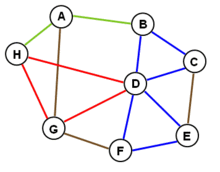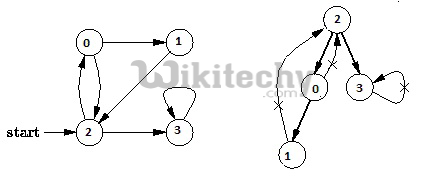Graph Cycle

# Detect Cycle in a directed graph using colorsDetect Cycle in a directed graph using colors-Graph cycle-Depth First Traversal can be used to detect cycle in a Graph. DFS for a connected graph.

Given a directed graph, check whether the graph contains a cycle or not. Your function should return true if the given graph contains at least one cycle, else return false. For example, the following graph contains three cycles 0->2->0, 0->1->2->0 and 3->3, so your function must return true.

Solution
Depth First Traversal can be used to detect cycle in a Graph. DFS for a connected graph produces a tree. There is a cycle in a graph only if there is a back edge present in the graph. A back edge is an edge that is from a node to itself (selfloop) or one of its ancestor in the tree produced by DFS. In the following graph, there are 3 back edges, marked with cross sign. We can observe that these 3 back edges indicate 3 cycles present in the graph.In the previous post, we have discussed a solution that stores visited vertices in a separate array which stores vertices of current recursion call stack.

In this post a different solution is discussed. The solution is from CLRS book. The idea is to do DFS of given graph and while doing traversal, assign one of the below three colors to every vertex.

```WHITE : Vertex is not processed yet.  Initially
all vertices are WHITE.

GRAY : Vertex is being processed (DFS for this
vertex has started, but not finished which means
that all descendants (ind DFS tree) of this vertex
are not processed yet (or this vertex is in function
call stack)

BLACK : Vertex and all its descendants are
processed.

While doing DFS, if we encounter an edge from current
vertex to a GRAY vertex, then this edge is back edge
and hence there is a cycle.
```

Below is C++ implementation based on above idea.

``````// A DFS based approach to find if there is a cycle
// in a directed graph.  This approach strictly follows
// the algorithm given in CLRS book.
#include <bits/stdc++.h>
using namespace std;

enum Color {WHITE, GRAY, BLACK};

// Graph class represents a directed graph using
class Graph
{
int V; // No. of vertices

// DFS traversal of the vertices reachable from v
bool DFSUtil(int v, int color[]);
public:
Graph(int V);  // Constructor

// function to add an edge to graph

bool isCyclic();
};

// Constructor
Graph::Graph(int V)
{
this->V = V;
}

// Utility function to add an edge
{
}

// Recursive function to find if there is back edge
// in DFS subtree tree rooted with 'u'
bool Graph::DFSUtil(int u, int color[])
{
// GRAY :  This vertex is being processed (DFS
//         for this vertex has started, but not
//         ended (or this vertex is in function
//         call stack)
color[u] = GRAY;

// Iterate through all adjacent vertices
list<int>::iterator i;
{
int v = *i;  // An adjacent of u

// If there is
if (color[v] == GRAY)
return true;

// If v is not processed and there is a back
// edge in subtree rooted with v
if (color[v] == WHITE && DFSUtil(v, color))
return true;
}

// Mark this vertex as processed
color[u] = BLACK;

return false;
}

// Returns true if there is a cycle in graph
bool Graph::isCyclic()
{
// Initialize color of all vertices as WHITE
int *color = new int[V];
for (int i = 0; i < V; i++)
color[i] = WHITE;

// Do a DFS traversal beginning with all
// vertices
for (int i = 0; i < V; i++)
if (color[i] == WHITE)
if (DFSUtil(i, color) == true)
return true;

return false;
}

// Driver code to test above
int main()
{
// Create a graph given in the above diagram
Graph g(4);

if (g.isCyclic())
cout << "Graph contains cycle";
else
cout << "Graph doesn't contain cycle";

return 0;
}``````

Output :

`Graph contains cycle`

Time complexity of above solution is O(V + E) where V is number of vertices and E is number of edges in the graph.

READ  Detect cycle in an undirected graph#### Venkatesan Prabu

Wikitechy Founder, Author, International Speaker, and Job Consultant. My role as the CEO of Wikitechy, I help businesses build their next generation digital platforms and help with their product innovation and growth strategy. I'm a frequent speaker at tech conferences and events.

X